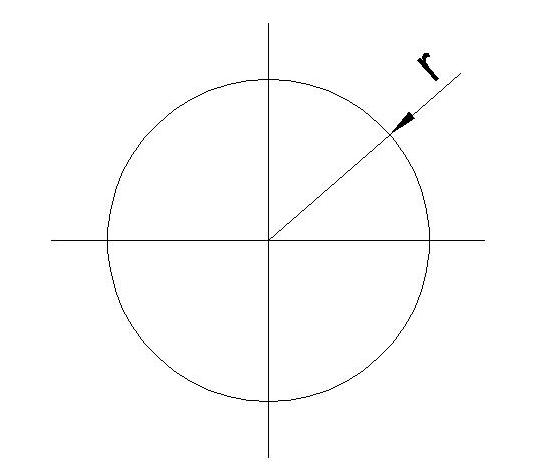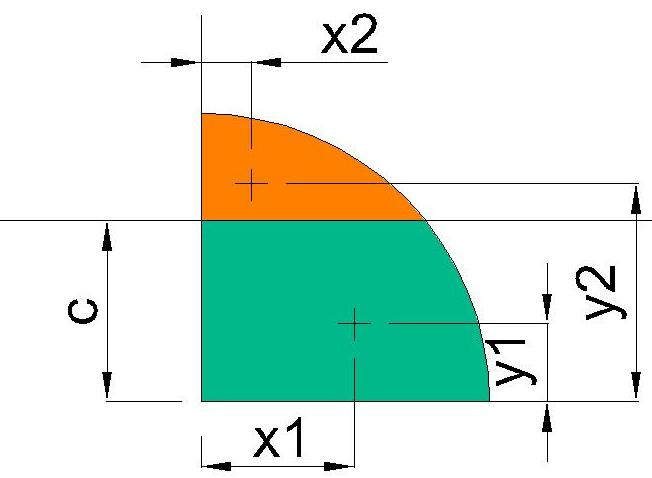# Geometric expressions for a quarter circle cut at an arbitrary point

## Homework Statement

I am after finding general geometric expressions for a quarter-circle that is split into two segments along either its domain or range (they are equal). I.e. Taking the circle shown in Figure 1 and concentrating on the upper right quadrant, I am after expressions for the individual areas (top and bottom) and their respective centroids x1, y1 and x2, y2 (Figure 2) when cut at "c".

Figure 1:Figure 2:## Homework Equations

Area of circle = ∏r$^{2}$

Equation of quarter circle: y(x) = √(x$^{2}$-r$^{2}$)

## The Attempt at a Solution

Apart from stating the obvious equations (above) I’m stuck on this problem.

I have searched my textbooks/google (using general key words) for expressions of this particular case with no luck.

I have never been strong with deriving expressions from first principals and would appreciate any ideas/push in the right direction. This is a problem directed at self-study, not homework.

$\bar{y}$=$\frac{4Rsin^{3}(\frac{1}{2}θ[STRIKE][/STRIKE])}{3(θ-sin(θ))}$
$\bar{y}$=$\frac{4R}{3π}$
A=$\frac{R^{2}}{2}$(θ-sin(θ))